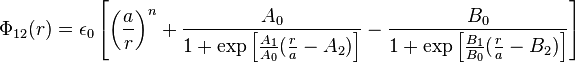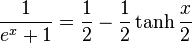2022: SklogWiki celebrates 15 years on-line

# Fermi-Jagla model$\Phi_{12}(r) = \epsilon_0 \left[ \left( \frac{a}{r} \right)^n + \frac{A_0}{1+\exp \left[ \frac{A_1}{A_0} (\frac{r}{a}-A_2) \right]} - \frac{B_0}{1+\exp \left[ \frac{B_1}{B_0} (\frac{r}{a}-B_2) \right]} \right]$$\frac{1}{e^x+1}=\frac{1}{2}-\frac{1}{2}\tanh \frac{x}{2}$